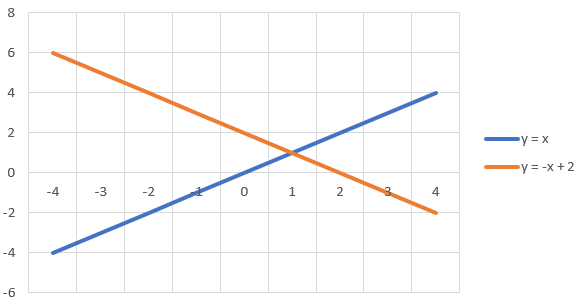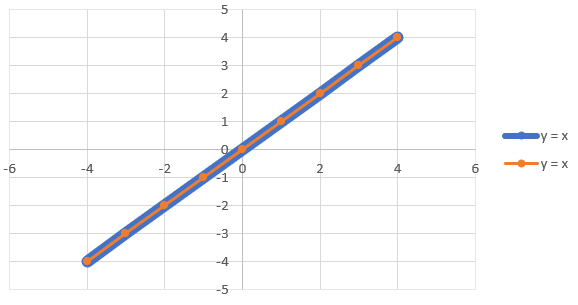# Linear Systems

A Linear System is a set of 2 or more Linear Equations. It is recommended you first view this lesson to better understand how linear equations work.

In a linear system, there are 3 unique cases for intersection:

1. Lines don't intersect; this means that they are parallel to one another:
2.3. Lines only intersect at one point; this is the most common form of linear system:
4.5. Lines intersect infinetely at every point; this means that the lines/equations are the exact same:
6.There are 3 main methods for solving a linear system:

## Graphing Method

• The simplest but least accurate way of portraying the relationships between the set of lines.
• All you need to do is graph the set of lines based on their resepctive equations to see if or where they intersect
• Before graphing the lines, we can create a table of values to identify where each point of the respective lines lie

Graph the lines $$y = 5x - 7$$ and $$y = -3x + 1$$ to find the Point of Intersection.

## Substitution Method

Example

Using the substitution method, we can solve the linear system with linear equations $$8x - y = 10$$ and $$3x - y = 9$$

First, solve one linear equation for $$x$$ in terms of $$y$$:

$$8x - y = 10$$
$$y = 8x - 10$$

Next, substitute that expression for $$y$$ in the other linear equation:

$$3x - (8x - 10) = 9$$
$$3x - 8x + 10 = 9$$

Solve this and you have the $$x$$-coordinate of the Point of Intersection:

$$3x - 8x = 9 - 10$$
$$-5x = -1$$
$$\cfrac{\cancel{-5}x}{\cancel{-5}} = \cfrac{-1}{-5}$$

$$x = 0.2$$

Plug $$x$$ ($$0.2$$) into either equation to determine the corresponding $$y$$-coordinate and the Point of Intersection:

$$8(0.2) - y = 10$$
$$1.6 - y = 10$$
$$- y = 10 - 1.6$$
$$-y = 8.4$$
$$\cfrac{-y}{-1} = \cfrac{8.4}{-1}$$

$$y = -8.4$$

Therefore, we can determine that the Point of Intersection is $$(0.2, -8.4)$$.

You can also start by solving for $$x$$ in terms of $$y$$ using the same steps outlined above.

## Elimination Method

Example

Using the elimination method, we can solve the linear system with linear equations $$4 + y -3x = 0$$ and $$x + y = 8$$

First, align all the terms in both equations so they share the same form (ie slope-int form):

$$y = 3x - 4$$
$$y = -x + 8$$

Add or subtract a multiple of one equation to (or from) the other equation so that the terms cancel each other out:

$$3(y = -x + 8)$$
$$3y = -3x + 24$$
$$(3y + y) = (-3x + 3x) + (24 - 4)$$
$$4y = 20$$
$$\cfrac{\cancel{4}y}{\cancel{4}} = \cfrac{20}{4}$$

$$y = 5$$

Next, solve for $$x$$ by substituting the $$y$$-value ($$5$$) into one of the original equations:

$$4 + 5 -3x = 0$$
$$-3x = -9$$
$$\cfrac{\cancel{-3}x}{\cancel{-3}} = \cfrac{-9}{-3}$$
$$x = 3$$

Therefore, we can determine that the Point of Intersection is $$(3, 5)$$.

Find the Point of Intersection between the linear equations $$y = 7x + 3$$ and $$x - y = 3$$ using any algebraic method.